407 493 6601

# Precalculus - The Law of Cosine and Area

## Introduction

• A triangle in which none of its interior angles are $90°$ is called a non-right triangle or oblique triangle.
• Since trigonometric ratios can only be applied to right triangles, how can we solve non-right triangles? [Note that solving a triangle means finding the measurement of its angles and sides].
• Here comes the role of the law of cosines. It relates the sides and angles of non-right triangles.

## The Cosine Rule

• Consider a triangle ABC as shown in the figure below:• The Law of Cosines or Cosine Rule states that:

${a}^{2}={b}^{2}+{c}^{2}-2bc·\mathrm{cos}\left(A\right)$

${b}^{2}={a}^{2}+{c}^{2}-2ac·\mathrm{cos}\left(B\right)$

${c}^{2}={a}^{2}+{b}^{2}-2ab·\mathrm{cos}\left(C\right)$, where

$a=$the side opposite to angle A

$b=$the side opposite to angle B

$c=$the side opposite to angle C

• The Cosine rule is applied under the following two conditions:

(a) when two sides and one included angle (SAS) is given, or

(b) when all three sides (SSS) are given.

## Area of a Triangle

There are several ways to calculate the area of a triangle depending on the dimensions of the given triangle.

Case (1): Finding the area of a triangle when we know the measure of two of its sides and the included angle between them.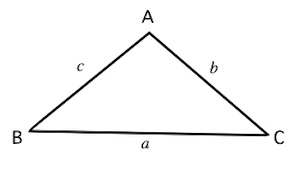• When the sides a and b and the included angle C are known, the area of the triangle can be calculated using the formula:

• When the sides b and c and the included angle A are known, the area of the triangle can be calculated using the formula:

• When the sides a and c and the included angle B are known, the area of the triangle can be calculated using the formula:

Case (2): Finding the area of a triangle when we know the measure of all its sides (Heron's Formula).

• When the three sides of a triangle a, b, and c are known, the area of the triangle can be calculated using the formula:

, where $s=\frac{a+b+c}{2}$ (semi-perimeter of the triangle)

## Solved Examples

Example 1: In $∆ABC$,  and . Find the measure of side $AB$.

Solution: Here, two sides and included angle between them (SAS) are given. Let us draw the triangle based on the given data.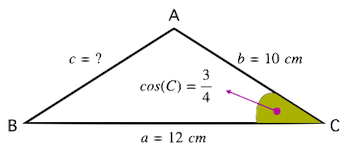Applying the Cosine rule in the given triangle:

or, ${c}^{2}={\left(12\right)}^{2}+{\left(10\right)}^{2}-2×\left(12\right)×\left(10\right)×\left(\frac{3}{4}\right)$

or, ${c}^{2}=144+100-180=64$ $⇒c=\sqrt{64}=8$

Therefore, the measure of side AB is .

Example 2: In $∆ABC$,  and . Find the measure of $\angle A$.

Solution: Here, three sides are given (SSS). Let us first draw a triangle with the given data.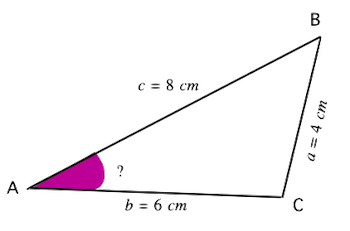Applying the Cosine rule in the given triangle:

or,

or, $=\frac{7}{8}$

$⇒\angle A={\mathrm{cos}}^{-1}\left(\frac{7}{8}\right)\approx 28.95°$

Example 3: Find the area of the triangle shown in the figure.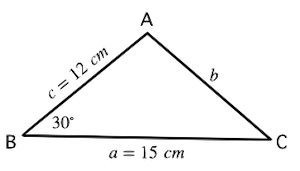Solution:

Example 4: Find the area of the triangle shown in the figure.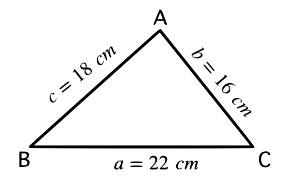Solution: , and

$s=\frac{a+b+c}{2}$ $=\frac{22+16+18}{2}$

$Are{a}_{△}=\sqrt{s\left(s-a\right)\left(s-b\right)\left(s-c\right)}$

$=\sqrt{28\left(28-22\right)\left(28-16\right)\left(28-18\right)}$

$=\sqrt{28×6×12×10}$

## Cheat Sheet

• For any triangle ABC (acute, obtuse, or right), if $a$$b$ and $c$ are the lengths of three sides opposite to $\angle A$, $\angle B$ and $\angle C$, respectively, then as per the law of Cosines or Cosine rule, the sides and angles of the triangle are related as mentioned below:

${a}^{2}={b}^{2}+{c}^{2}-2bc·\mathrm{cos}\left(A\right)$

${b}^{2}={a}^{2}+{c}^{2}-2ac·\mathrm{cos}\left(B\right)$

${c}^{2}={a}^{2}+{b}^{2}-2ab·\mathrm{cos}\left(C\right)$

• When the law of cosines is applied to a right triangle, the result is the Pythagorean theorem.
• The area of a triangle is half the product of the measure of any of its two sides times the sine of the included angle between the given sides.
• When all the sides of a triangle are given, the area of the triangle can be computed using the following formula:

${\text{Area}}_{△}\text{=}\sqrt{s\left(s-a\right)\left(s-b\right)\left(s-c\right)}$, where $s=\frac{a+b+c}{2}$

## Blunder Areas

• The Laws of Cosines can be used in any triangle (not just right-angled triangles) except when there is ambiguity.